# Square shoulder + square well model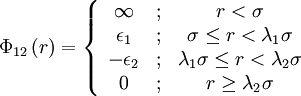$\Phi_{12}\left( r \right) = \left\{ \begin{array}{ccc} \infty & ; & r < \sigma \\ \epsilon_1 & ; &\sigma \le r < \lambda_1 \sigma \\ - \epsilon_2 & ; &\lambda_1\sigma \le r < \lambda_2 \sigma \\ 0 & ; & r \ge \lambda_2 \sigma \end{array} \right.$
where$\Phi_{12}(r)$ is the intermolecular pair potential,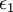$\epsilon_1$ and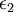$\epsilon_2$ are the well depths,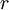$r$ is the distance between site 1 and site 2 where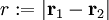$r := |\mathbf{r}_1 - \mathbf{r}_2|$, σ is the hard core diameter and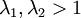$\lambda_1,\lambda_2>1$